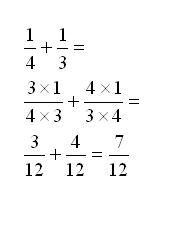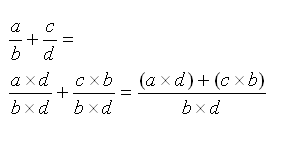# Scratch ProgrammingScratch Maths

## Introduction

You can write programs in Scratch not just to solve problems in Maths but also to help you understand the problems too.

This page contains a range of programming tasks that support a variety of topics in Maths. For each topic, a link to BBC Bite Size Revision pages has been provided to remind you of the details of the topic.

## Percentages

### Concepts

• Convert a fraction to a percentage.
• Find x% of a quantity

BBC Bite Size Percentages Revision

### Fraction To Percentage

``` If there are 80 pupils and 13 of them have black hair, what percentage of the students has red hair? Percentage of pupils with black hair = ( 13 ÷ 80 ) x 100 The general rule for this is: ( a ÷ b ) x 100 where a is the number of people we want to count and b is the total number of people. ```

### X% Of A Quantity

``` 23% of 75 = ( 23 ÷ 100 ) x 75 The general rule for this is: a% of b = (a ÷ 100) x b where a is the percentage you want to find and b is the number you want to find it from. ```

## Probability

### Concepts

• Finding the probability of an outcome
• Comparing calculated probability with experimental probability

BBC Bite Size Probability Revision

### Finding The Probability Of An Outcome

``` Probability = Number of ways outcome can happen ÷ Total number of outcomes ```

### Comparing Calculated Probability With Experimental Probability

``` You can set up simulations in Scratch with random numbers. Set up a program with variables called heads, tails and thisthrow. Set all of the variables to 0. Use a Repeat block to make the next section repeat, say 100 times. Set thisthrow to a random number from 1 to 2. If the number is 1 change heads by 1, if the number is 2 change tails by 1. Your calculated probability for these events would suggest roughly the same number of heads and tails. Repeat the experiment with different numbers of throws. How high does the number have to get for the two totals to be close to the same value. Repeat the experiment for the rolling of a 6-sided die. ```

## Mean Average

### Concepts

• Finding the mean of a set of numbers
• Finding the largest and smallest numbers

BBC Bite Size Mean Revision

### Calculating The Mean

``` Mean = Sum Of Numbers ÷ Amount Of Numbers Your program should allow the user to enter as many numbers they want, the program has to keep a running total of what is entered as well as a count of the amount of numbers entered. You will need 3 variables - mean, count and thisnumber. Set the variables to 0 at the start. Create a loop that repeats until thisnumber=end. Inside the loop, ask the user to enter a number or 'end' to stop. If they don't enter 'end', change the count by 1 and change the total by thisnumber After the loop, set the mean to be the total ÷ count and output the value. ```

### Finding The Largest & Smallest Values

``` You can add this to the code you wrote for the previous program. Create two more variables minimum and maximum and set them to be equal to 'nothing' at the start of the program. Inside the loop add some If blocks. If minimum = nothing Then set minimum to thisnumber If maximum = nothing Then set maximum to thisnumber If thisnumber < minimum Then set minimum to thisnumber If thisnumber > maximum Then set maximum to thisnumber Finally, output the minimum and maximum values along with the mean after the loop. ```

The difference between the largest and smallest numbers is the range of the numbers entered.

## Factors & Prime Numbers

### Concepts

• Listing the factors of a number
• Determining if a number is prime

### Listing Factors

Here is a list of text instructions that you can convert into a program that finds all of the factors of a number. A factor of a number is a number that divides into that number without any remainder.

```Ask for the number Set number variable to what was entered Set factors variable to 1 and a space joined together Set counter variable to 2 Repeat number - 1 times    If number mod counter = 0 Then       Set factors to Join (factors, counter, space)    End If    Change counter by 1 End Repeat Output factors```

### Determining If A Number Is A Prime Number

Here is a list of text instructions that you can convert into a program that finds out if a number is prime - ie that it has only itself and 1 as factors.

```Set prime variable to true Set numbertocheck variable to a number entered by the user Set counter variable to 2 Repeat until counter > numbertocheck ÷ 2 OR prime = false    if numbertocheck Mod counter = 0 then       Set prime to false    End If    Change counter by 1 End Repeat If prime=true Then    Say number is prime Else    Say number is not prime End If```

As an extra challenge, you can convert your second program to tell you all of the prime numbers up to 1000. You will need an extra loop to do this and you should change the output to only output numbers if they are prime.

## Fractions

This is one of the more challenging Maths topics to program. It's also one of the most useful.

### Concepts

• Finding the highest common factor of two numbers
• Writing a fraction in its simplest form
• Converting improper fractions to mixed number form and back again
• Multiplying and dividing fractions

BBC Bitesize Fractions Revision

### Finding The Highest Common Factor Of Two Numbers

Although not strictly about fractions, being able to calculate the HCF of two numbers is needed to be able to write a fraction in its simplest form. There are many ways to do this and they're all a bit tough to follow. Try the following instructions and test your program very well to make sure it works.

```Set numerator variable to what the user enters Set denominator variable to what the user enters Set variable a to numerator Set variable b to denominator Repeat Until a = b    If a>b Then       Set a to a-b    End if    If b>a Then       Set b to b-a    End if End Repeat Set hcf variable to a Output hcf```

### Writing A Fraction In Its Simplest Form

You will need to have completed the highest common factor program to be able to do this.

```Ask user to input numerator and denominator Work out the highest common factor of these two numbers Divide both numbers by the highest common factor Output the fraction```

### Improper Fractions

An improper fraction is one where the numerator is larger than the denominator. For example, seven fifths - 7/5. Normally, these fractions are output in mixed number form, for example 7/5 = 1 2/5.

```Set wholenumber variable to numerator ÷ denominator Set wholenumbervariable to round (wholenumber - 0.5) Set numerator to numerator Mod denominator Output the new fraction```

You should be able to write code to reverse the process too.

To be able to add two fractions together you need to have the same denominator. Here is an example of how you might add together two fractions,If we rewrite these calculations using letters instead of numbers, we get,From this we can see two formulas for calculating the numerator and denominator of the result of the addition.

A similar process is followed for subtracting fractions, replace the addition signs with subtraction signs.

### Multiplying & Dividing Fractions

These two operations are more straightforward than addition and subtraction because you don't need to find an HCF or LCM to do the calculation. Click the Bite Size link above and remind yourself of how to perform the calculations - then have a go at writing the programs.

### Fraction Frenzy

One last job would be to tie up all of the different fraction programs into one big fraction calculator than you can use to check answers to Maths problems.